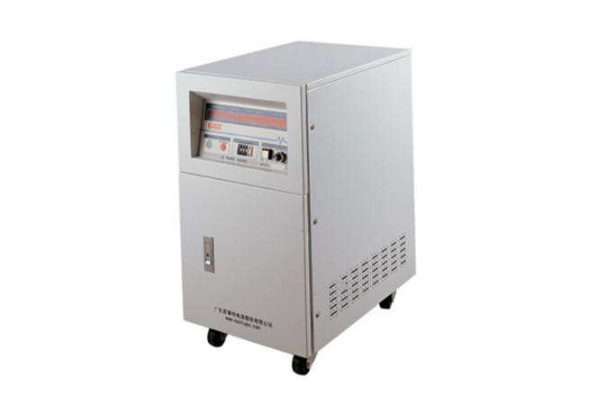Home >> Knowledge >>
The difference between variable frequency power supply and power supply
1.Difference between variable frequency power supply and power supply – Purpose

Frequency conversion power supply by changing the output power voltage and frequency, and provide a pure stable power supply, for the use of the load, and the frequency converter is by changing the frequency and voltage, to achieve the motor slow start function, voltage and frequency change in proportion, can not be adjusted separately.

2. Difference between variable frequency power supply and power supply -- Load type
Variable-frequency power supply is suitable for resistance, capacitance, sensibility and other loads. The frequency converter is mainly used for precise control of the load speed of motor and cannot be used for other loads and tests.

3. Difference between variable frequency power supply and power supply--output voltage
The output voltage of the inverter power supply under full load condition, the output voltage stability is 1% of the set voltage, and the output frequency of the converter changes with the change of the motor operating characteristics.

4. Difference between variable frequency power supply and power supply -- output frequency
As for the output frequency, in the case of full load, the output frequency stability of the frequency conversion power is 0.01% of the set frequency, and the output frequency of the frequency converter changes with the change of the motor's working characteristics.

5. Difference between variable frequency power supply and power supply -- output waveform
In addition, the output waveform of the variable frequency power supply is pure sinusoidal square wave, while for the converter, the output waveform distortion is less than 2% under full load, and the waveform distortion is at least 30%.SUKI
2020-10-15

声明： Disclaimer: This article comes from individual, KRECO has the right of final interpretation.